# Probability of Dependent Events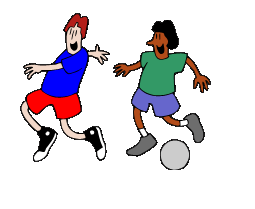Dependent Events: Imagine that you are one of the
captains forming a kickball team. The other captain chooses
Lisa, the player that you were about to choose. So when
it comes to your chance to choose, you have to
pick someone else.

Your choice depended on the selection of the other captain. This is an example of a dependent event.

Let's look at some examples and check out the math involved.

Examples:

1.  Cara has a bag of marbles. There are 15 total. 7 are white, 3 are green, 2 are
blue and 3 are purple.

a. What is the probability of choosing a white and then a green,
without replacement?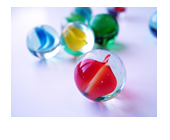Because the first marble is NOT being replaced, the probability of the second marble depends on the first. When the second marble is chosen, there are only 14 total marbles instead of 15, because one of the marbles has been removed.

Step 1: Determine the probability of the first marble being white.Step 2: Determine the probability of the second marble being green.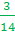Step 3: Multiply the probabilities together to determine the probability of both events occurring.There is a 10% chance of grabbing a white and then a green.

b. Determine the probability of choosing a blue and then a purple marble if the first marble is NOT replaced.

Step 1: Determine the probability of the first marble being blue.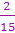Step 2: Determine the probability of the second marble being purple.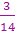Step 3: Multiply the probabilities together to determine the probability of both events occurring.There is about 3% chance of grabbing a white and then a green.

What is the chance of Dennis reaching in and eating a sugar cookie and then a chocolate chip cookie?

Because Dennis will eat one of the cookies, the chance of the second cookie being a certain type depends on the first choice. This is an example of a dependent set of events.

Also note that there are 25 total cookies. 10 + 8 + 7 = 25

Step 1: Determine the probability of getting a sugar cookie.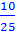Step 2: Determine the probability of getting a chocolate chip cookie.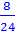Step 3: Multiply the probabilities together.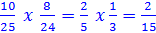There is about a 13% chance of Dennis eating the cookies in this order.

Let's Review:

Dependent events occur when the probability of one event depends on what happened in the prior event. To calculate the probability, you would first determine the probability of each event and then multiply the probabilities together.